# Tangent properties

## What is a tangent of a circle

When you have a circle, a tangent is perpendicular to its radius. It touches (intersects) the circle at only one point and looks like a line that sits just outside the circle's circumference. The fact that it is perpendicular will come in useful in our calculations as we can then make use the Pythagorean theorem.

## How to find the tangent of a circle

In the questions you'll have to answer in this lesson, you'll either be given the tangent or you'll have to look for it. Some tangent properties that you should keep in mind to help you solve problems include:

1) A tangent is perpendicular to the radius at the point of tangency.

2) Tangent segments to an external point of a circle are equal.

3) The angle between a tangent and a chord is equal to the inscribed angle on the opposite side of that chord.

You'll see these properties in use in the practice problems coming up when dealing with a (or several) tangent line on a circle.

## What is a chord in a circle

In a circle, a chord is a line that has both endpoints lying on the circle. A chord has several properties and some of them are:

1) If two chords' lengths are equal in a circle, they are equidistant from the center.

2) The longest chord in a circle is the diameter and it passes through the center of a circle.

3) A perpendicular bisector of a chord passes through the center of a circle.

Understanding chords will help you tackle problems dealing with tangents.

## Example problems

Question 1: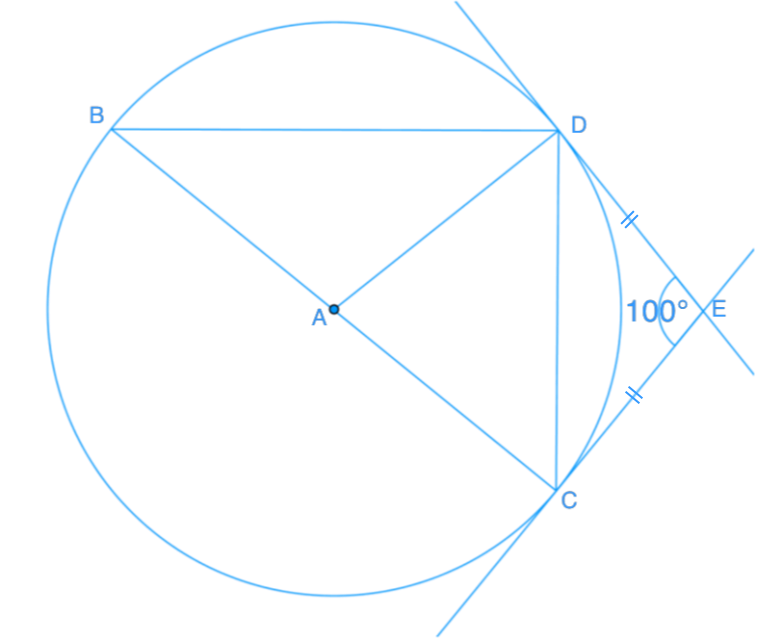Tangent properties and angles in a circle

Find angle CBD

Solution:

First, look for useful information from the graph:

Triangle CDE is an isosceles triangle.

Angle CED is 100°. Therefore angle CDE and angle DCE = 40°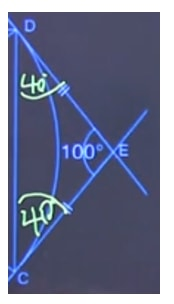Find out that the angle CDE and DCE = 40°

Point A is the center of the circle.

Point D and point C are point of tangency to the circle. Line AD and line AC are perpendicular to the tangent line. Therefore, Angle ACE = 90°.

Angle ACD = Angle ACE – Angle DCE = 90° - 40° = 50°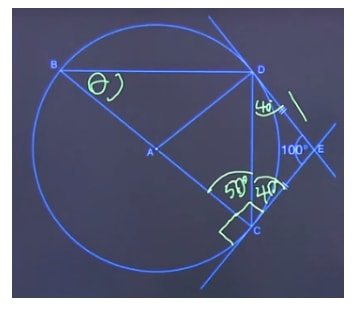Calculate angle ACE by ACE - DCE

Angle CDB is an inscribed angle of the diameter. So, Angle CDB = 90°.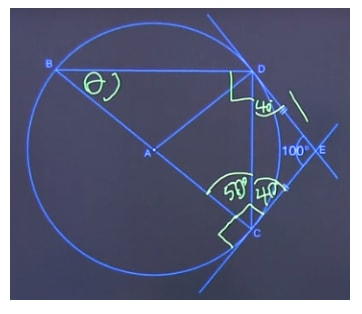CDB = 90° because of inscribed angle of diameter

angle CBD = 180° - Angle ACD - Angle CDB = 180° - 50° - 90° = 40°

Question 2:

In the diagram below, BC is a diameter, and AB is tangent to the circle at point B. OB = 6 cm, and AB = 8 cm.

Find the length of the chord DC.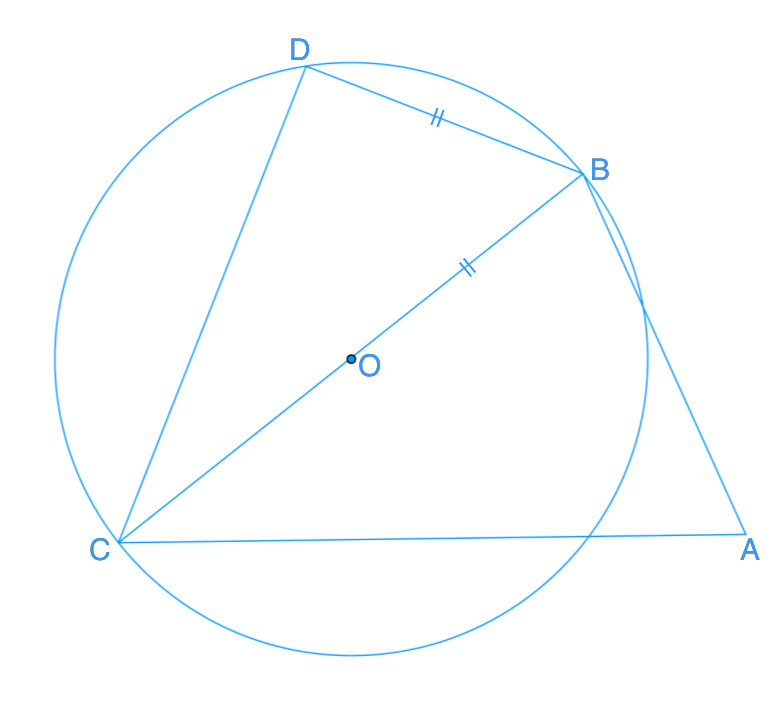Using tangent properties to find missing angles and lengths

Solution:

OB is the radius = 6 cm. So, the diameter BC = 12 cm

OB = DB – 6 cm

Angle CDB = 90° because it is an inscribed angle of the diameter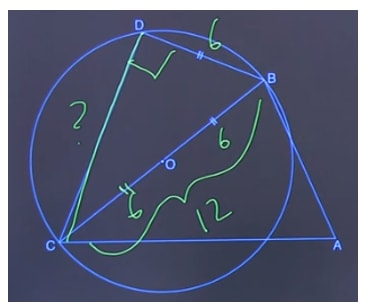Angle CDB = 90° because of inscribed angle of the diameter

Using the Pythagorean theorem:

$c^{2} = a^{2} + b^{2}$

$12^{2} = 6^{2} +b^{2}$

$\sqrt{12^{2} - 6^{2}} = b$

$b = \sqrt{108}$

$b = 10.39cm$

Question 3

Find the length of ACUsing tangent properties to find missing angles and lengths

Solution:

AB is tangent to the circle. So angle ABC = 90°

OB is the radius = 6 cm. So, the diameter BC = 12 cm

AB = 8cm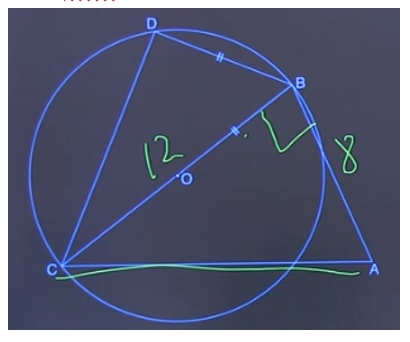Find the length of diameter and BC

Using the Pythagorean theorem:

$c^{2} = a^{2} + b^{2}$

$c = \sqrt{12^{2} + 8^{2}}$

$c = \sqrt{80}$

$c = 8.94cm$

Check out this online calculator to help you figure out the lengths of the tangents of a circle. Ready to build off on this lesson? Learn about the arcs of a circle, area of a circle, and central and inscribed angles.

### Tangent properties

A tangent to a circle must form a perpendicular line from the point of tangency to the center of the circle. In this lesson, we will learn how to use this property, along with others such as, chord properties, to solve questions.

#### Lessons

• Introduction
a)
1. What is a chord?
2. What is a tangent?

b)
What are the properties of a tangent?

• 1.Find.
a)
$\angle$DCE

b)
$\angle$CBD

c)
$\angle$BAD

• 2.
In the diagram below, BC is a diameter, and AB is tangent to the circle at point B. OB = 6 cm, and AB = 8 cm.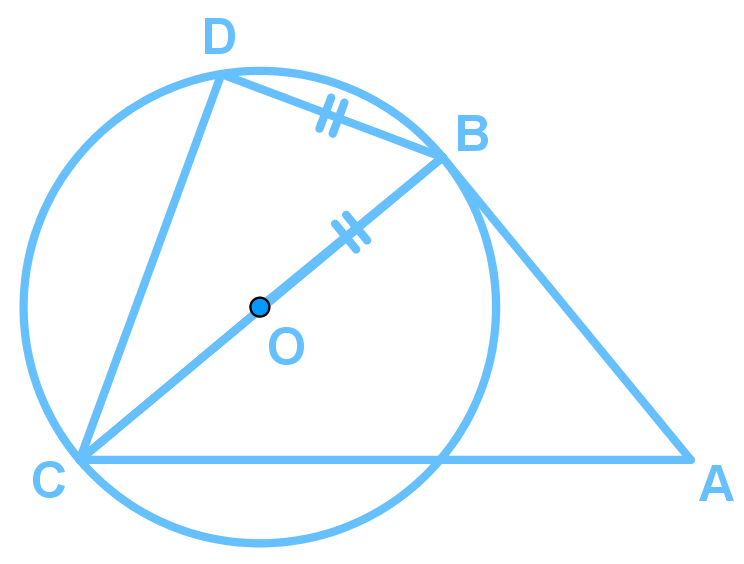a)
Find the length of the diameter.

b)
Justify if $\triangle$CBD is a right angle.

c)
Find the length of the chord DC.

d)
Find $\angle$ABC.

e)
Find the length of AC.

f)
If there is a straight line connecting point D and point O, what type of triangle is $\triangle$BOD?

• 3.
The furthest the hammer can travel in a hammer throw game is when the hammer travels along a tangent. If a hammer lands 62 m away from the athlete, how far did the hammer travel along a tangent? (Hint: The hammer is 1.2 m in length.)